## Abstract

Using cold EGR method with variable venturi and turbocharger has very significant effect, simultaneously on the reduction of NOX and grime simultaneously. EGR cooler is one of the most important parts in the cold EGR circuit. In this paper optimum design of cooler for working in different percentages of EGR, besides determination of optimum temperature of exhausted gases, efficiency growth, weight reduction, dimension and expenditures reduction, sediment reduction, and optimum performance by using gasoil which has significant amounts of brimstone, is investigated and optimized.

## Keywords

Cold EGR; NOX; Cooler

## Nomenclature

Ato- total heat transfer area (m2)

Ao- external heat transfer area of tube (m2)

Ai- internal heat transfer area of tube (m2)

Aw- logarithmic mean heat transfer area (m2)

Cps- specific heat of shell fluid (cooler fluid) (J/kg K)

Cp- specific heat of gas (J/kg K)

De- equivalent hydraulic diameter (m)

Di- tube internal diameter (m)

Do- tube external diameter (m)

Ds- shell internal diameter (m)

F- correction factor of LMTD at multi pass flows

hid- heat transfer coefficient of shell in ideal flow model (W/m2 K)

ho- heat transfer coefficient in shell side (W/m2 K)

ht- heat transfer coefficient in tube side (W/m2 K)

Jl- correction factor for effects of wall seals

Jb- correction factor for cross flows

Js- correction factor for walls distance at inlet and output sections

Jr- correction factor for Reynolds number less than 100

Jc- correction factor for fluid shear effect near the wall

kt- thermal conductivity of tube side fluid (W/m K)

kw- thermal conductivity of wall (W/m K)

Nt- number of tubes

NTU- number of heat transfer unit

${\textstyle {\overset {\cdot }{m}}_{S}}$- mass flow rate of shell side fluid

Q- actual heat transfer rate (W)

Uo- overall heat transfer coefficient (W/m2 K)

Ri- tube internal fouling resistance (m2 K/W)

Ro- tube external fouling resistance (m2 K/W)

tw- thickness of tube wall (m)

${\textstyle {\mu }_{s}}$- cooling fluid viscosity (water) (N s/m2)

${\textstyle {\mu }_{t}}$- hot fluid viscosity (gas) (N s/m2)

${\textstyle \epsilon }$- exchanger effectiveness

${\textstyle \varphi }$- temperature dependent viscosity correction factor

## 1. Introduction

The most important pollutants of diesel engines are Nitrogen Oxides (NOX) and grime particles. One of most effective methods for NOX pollutants reduction is restoring exhausted gases (EGR) into the combustion chamber; this action would be possible by three following methods: Thermal, Chemical and Dilution .

By cooling EGR gases (Cold EGR), more percent of exhausted NOX pollutants will be decreased. In cold EGR, restored gases are cooled by a heat exchanger and amount of absorbed heat by EGR will be increased  and . In other side, by decreasing the restored gases temperature, durability of EGR valves and other parts which are depended on EGR will be increased.

In diesel engines, most of produced Nitrogen Oxides are thermal, and by increasing combustion temperature, the rate of NO production will be higher. In other side, carbon suspended particles are the results of imperfect combustion and reduction of temperature. However, the most important effort of pollutants reduction and estimating new environmental standards in diesel engines is to reduce Nitrogen Oxides and grime simultaneously.

For diminishing Nitrogen Oxides and particles with no brunt to fuel economics, engine systems should be designed and controlled carefully to acquire optimum conditions of inlet temperature and pressure, time and amount of fuel injection, and quantity and schedule of input EGR. Prediction of all actual optimization variables is a very complicated and important obligation.

Numerous experiences on different EGR systems of heavy diesel engines, in scope of measuring performance affections, special fuel consumption and pollution, reveal that cold EGR (synthesis of high pressure exhausted gases and high pressure input air) or in other words short circuit EGR method with a variable venturi, with increased injection pressure (common rail) and turbocharger using, in comparison with the low pressure EGR system (synthesis of hot exhausted gases and input air before turbocharger), or in the other words long circuit EGR system, is more effective for NOX reduction and obtaining minimum amount of increased specific fuel consumption. Also short circuit EGR obstructs sediments in compressor and intercooler (if there is); in other side retardation of injection, increases the specific fuel consumption  and . Fig. 1a shows these two systems.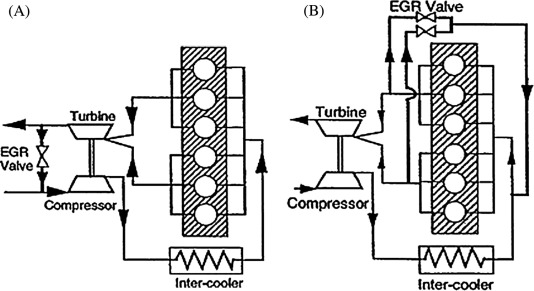Figure 1. (A) High pressure EGR system and (B) low pressure EGR system.

Briefly about reduction of NOX and grime in cold EGR, we can mention two important factors: firstly input load temperature reduction, lengthens combustion retardation and consequently induces more homogeneity between fuel steam and Oxygen synthesis; and secondly trapped air mass in the cylinder in IVC will increase which induces λ increment and oxygen concentration. Input Oxygen density increment will reduce dilution effects. Also in this situation cylinder pressure increment and consequently fortification of compress and combustion stroke cause effective pressure optimization which will optimize fuel consumption.

One of important sections of cold EGR design is designing and manufacturing of heat exchanger for gas cooling, which since now will be called EGR cooler. EGR coolers are mostly in shell-tube type ,  and ; in this case gas flows through tubes and coolant (engine jacket water) flows into the shell. This facilitates tubes cleanings and controlling of cooler exhausted gas temperature.

Inspected results reveal that although tubes numbers increment will increase the shell dimension (which will decrease heat transfer coefficient), in other side will increase heat exchanger and heat transfer area and decrease pressure-drop . However, tubes numbers increment will increase weight and production expenditures.

By using passive heat transfer methods such as spiral tubes we can increase heat transfer efficiency and decrease the length of heat exchanger . Fig. 2 shows four types of enhanced tubes and simple tubes, and compares them with regard to rate of heat transfer, pressure-drop and manufacturing cost . Also a specimen of spiral tubes is shown in Fig. 3. About heat transfer increment, spiral tubes have more efficiency in comparison with other extended tubes; also they have appropriate production cost and pressure-drop.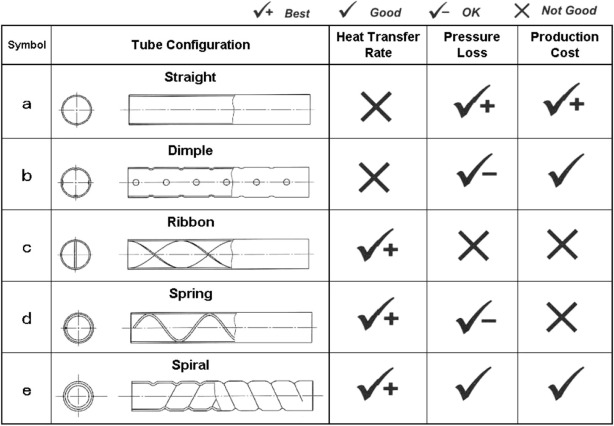Figure 2. Comparison of different tubes which are used in heat exchangers .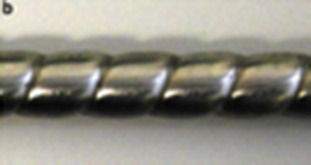Figure 3. Specimen of spiral tube .

Using spiral tubes will be very effective in scope of equalizing fluid speed in the shell, local boiling and thermal stresses obstruction, and intensifying perpetuity of cooler .

Pressure-drop at the gas, and subsequently speed decrement of it in tubes, is a design restrictive factor. With regard to EGR flow different percentages, Reynolds number ranges between 2000 and 9000 at the cooler; it is between transient flow ranges which does not have precise figured results for calculating heat transfer coefficient. So estimating friction coefficient by using analytic methods will not get a trusted quantity.

Also we cannot calculate actual pressure-drop during entrance diffuser. Moreover a stagnation pressure point will be created at return section. This happens at the end of diffuser, flow striking section to the wall, and boundary layer contact at conical section; which has a very important effect on the amount of crossing flow, particularly at side tubes. Therefore in scope of investigation of diffuser entrance diameter effects on the uniform and effective distribution of returning gases into all tubes, and cooler performance estimation, some calculations are done by CFD method.

Significant portion of pressure-drop in diffuser is due to flow shear stress at returning section.

Despite rational pressure-drop, appropriateness with working conditions gets designed cooler to have higher efficiency in comparison with typical coolers ,  and .

## 2. Equations and solution methods

Governing equations encompass continuity, momentum, energy, correlated pressure-drop and heat transfer relations. Applying different EGR percentages and presence of various design parameters such as arrangements, sizes, number of passages, and type of tubes leads us to use trial and error method which brings complexity in design; therefore, a code is written in Fortran 90.

Elementary design for different EGR percentages firstly is done by LMTD method and tubular exchanger manufacturers’ association standard (TEMA) and then is probed and proved by   the number of transfer units’ ${\textstyle \left(\epsilon -NTU\right)}$ method. Also permissive amount of pressure-drop is investigated by related equations in the tubes.

In the LMTD method heat transfer area is calculated by the following equation :

 $A_{to}={\frac {Q}{U_{o}F\Delta T_{LN}}}$
(1)

${\textstyle \Delta T_{LN}}$ is LMTD of Counter flow and is described as follows:

 $\Delta T_{LN}={\frac {\left(T_{h1}-T_{c2}\right)-\left(T_{h2}-T_{c1}\right)}{ln\left((T_{h2}-T_{c1})/\left(T_{h1}-T_{c2}\right)\right)}}$
(2)

Major calculations in EGR cooler design are related to Uo calculation; this coefficient is depended on fouling resistance and heat transfer coefficients of shell and tube sides.

 ${\frac {1}{U_{o}}}={\frac {1}{1/h_{o}+A_{i}R_{i}/A_{o}+t_{w}/k_{w}A_{w}+A_{i}/A_{o}h_{t}+R_{o}}}$
(3)
 $A_{w}={\frac {D_{o}-D_{i}}{ln\left(D_{o}/D_{i}\right)}}$
(4)

In cooler tubes, flow is in transient conditions and there is no precise solution for heat transfer coefficient calculation. One of the best graphs to obtain this coefficient in tubes is Tate & Sieder which is shown in Fig. 4.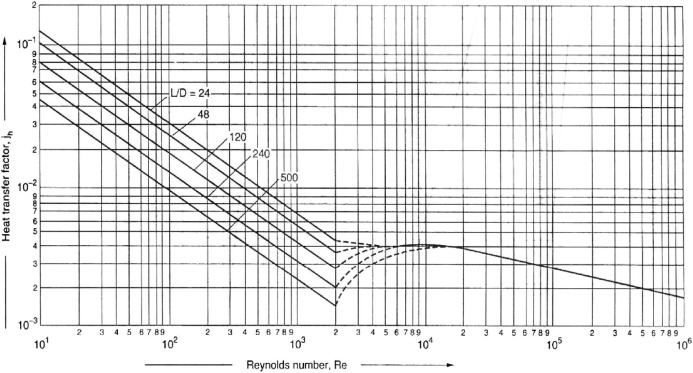Figure 4. Heat transfer coefficient in the tube by Tate & Sieder .

We can arrive to the following relations by fitting this curve in this section for different proportions of L/D, where L is the length of tube and D is the internal diameter of tube.

 $J_{H}=0.0044Re-(2.363Ln(L/D_{e})-6.46)\quad 2100\leqslant Re\leqslant 10000$
(5)

hi is heat transfer coefficient in tube and is acquired by following equation:

 $J_{H}={\frac {h_{i}D_{e}}{k}}{\left({\frac {c_{P}\mu }{k}}\right)}^{-1/3}{\varphi }^{-1}$
(6)

where

• JH is J-Colburn coefficient,
• ${\textstyle \varphi }$ is correction factor, depended on temperature, for viscosity.

In the ${\textstyle \left(\epsilon -NTU\right)}$ method effectiveness (${\textstyle \varphi }$) is described as follows:

 $\epsilon ={\frac {Q}{Q_{max}}}$
(7)
 $NTU={\frac {{AU}_{m}}{C_{min}}}$
(8)

Basic equation for mean heat transfer coefficient calculation in shell side is explained on the basis of Bell–Delaware method  as follows:

 $h_{o}=h_{id}J_{c}J_{l}J_{b}J_{s}J_{r}$
(9)

where hid   is heat transfer coefficient for completely cross flow with ideal tube set and ${\textstyle J}$’s are correction factors for seals and others:

 $h_{id}=j_{i}c_{ps}\left({\frac {{\overset {\cdot }{m}}_{s}}{S_{m}}}\right){\left({\frac {k_{s}}{c_{ps}{\mu }_{s}}}\right)}^{\frac {2}{3}}{\left({\frac {{\mu }_{s}}{{\mu }_{s{\mbox{,}}w}}}\right)}^{0.14}$
(10)

Factors calculations and complete information for heat transfer coefficient and pressure-drop calculations in shell and tube sides are mentioned in  and  references.

For figuring out number of tubes, if shell diameter is less than 230 mm following relations should be used. For triangular arrangement Eq. (11) and for quadrangular and turned quadrangular arrangement Eq. (12) are used.

 $N_{t}={\frac {3\lambda }{4}}\left(3{\frac {D_{S}-D_{o}}{2P}}\right)\left({\frac {D_{S}-D_{o}}{2P}}+\right.$$\left.1\right)+1$
(11)

 $N_{t}={\frac {3\lambda }{4}}{\left(0.886{\frac {D_{S}-D_{o}}{P}}+1\right)}^{2}$
(12)

For one and two tube passes ${\textstyle \lambda }$ is 1 and 0.92 respectively. Calculations reveal that number of tubes for triangular arrangement and circular arrangement is equal.

## 3. Simulation and results validation

With regard to problems such as transient flow in tubes, shortness of cooler length, and effects of entrance length, and what are mentioned before, usage of computational fluid dynamics method (CFD) for flow specimens estimation, temperature distribution and pressure in cooler, is very advantageous. Computational fluid dynamics software which is used in this paper is Fluent. In simulations pressure basis algorithms are used for continuous solving of converted differential equations in numerical form. Used algorithm here is SIMPLE. In every solving section, independence of solving trend from mesh form is assessed.

For numerical results validation, firstly a comparison between experimental data  and numerical results for a cooler tube simulation is done; then in scope of heat transfer and pressure-drop investigation, simulations for three entrance shapes for the whole cooler are probed. In Fig. 5 experimental and numerical results in tube length for two sections of center and 0.9 mm near the wall are exhibited. In this simulation, results are acquired for inlet gas at 250 °C and 0.25 g/s, in which temperature of cooler water is supposed to be permanent at 90 °C, for this condition acquired Reynolds number is 2000. Also exhausted gas specifications are supposed to be mean specifications of combustion productions.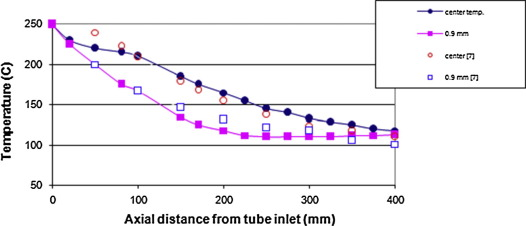Figure 5. Gas temperature diversion through a simple tube.

In next stage numerical analysis for cooler and three types of diffuser (cylindrical, nuzzle and conical) with flows of 100 g/s, 150 g/s and 200 g/s is done and compared with the experimental results. Results comparisons are shown in Fig. 6 in pressure-drop form, on flow basis, for a quarter prototype. Moreover, for more information, outlet temperature of cooler, related to flow rate, is shown in Fig. 7. Internal diameter of shell is 78 mm and tube number is 70. Chart shows a good coincidence between experimental and numerical results which maximum deviation is about 7%.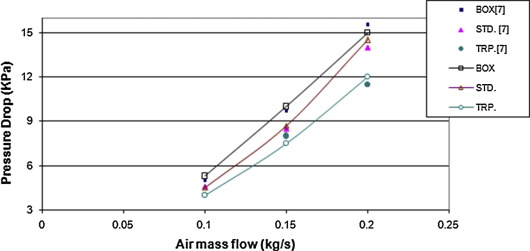Figure 6. Pressure drop related to flow rate.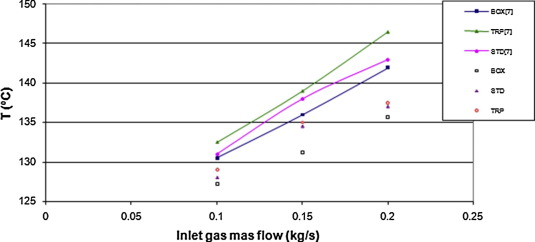Figure 7. Outlet temperature of cooler, related to flow rate.

Spiral tubes usage prompts growth of heat transfer efficiency in tube side and reduction of cooler size and weight. However, it will induce pressure-drop increment in tube side. Some optimizations are done for reducing this negative effect to an acceptable amount. Effective parameters of Spiral tube design on flow specifications and heat transfer, comprise tube pitch, spiral angle, spiral height, and spiral numbers.

As shown in Fig. 8, spiral wall induces fluid spinning near the wall; at this region fluid velocity exceeds plain tube velocity; also boundary layer is thinner than plain tube. Subsequently heat transfer coefficient near the wall is larger and as a result heat transfer rate is greater than before. Results are acquired for a tube with internal diameter of 7.8 mm, pitch of 1.4 mm, length of 200 mm and gas flow of 2.21 kg/h.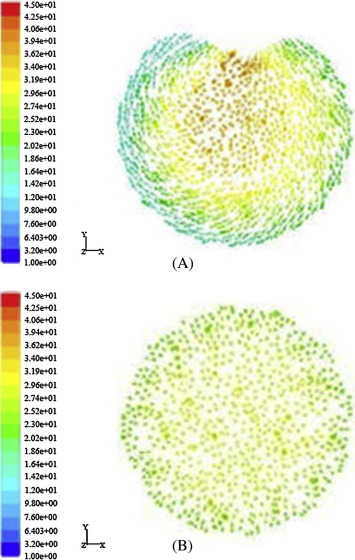Figure 8. A & B-velocity contours at plain tube and one way spiral tube.

With regard to negative effects of pressure-drop on heat transfer rate in cooler tubes, effects of spiral numbers increment on pressure-drop and heat transfer are probed. As shown in Fig. 9, increase of spiral numbers from one to two (or four), with constant hydraulics diameter, induces central flow symmetry and decreases fluid velocity in comparison with one spiral tube.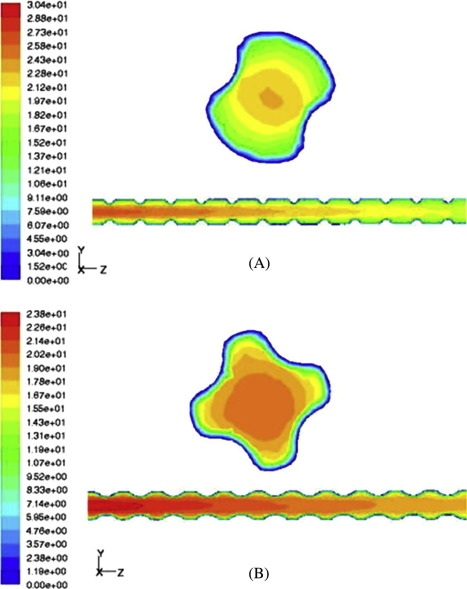Figure 9. A & B-velocity contours at 2 and 4 way spiral tube.

Also by increasing heat transfer area because of increment of spiral numbers significant decrement is not seen in thermal efficiency in comparison with one way spiral tube.

Obtained results are shown in Figure 10 and Figure 11.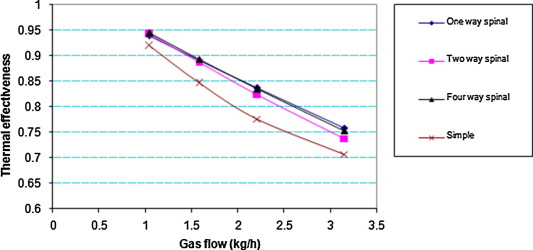Figure 10. Spiral number effect on thermal effectiveness.Figure 11. Spiral number effect on pressure drop.

Spiral pitch reduction and spiral height increment, cause heat transfer coefficient growth.

Sediments increment at the tube wall, decreases total heat transfer coefficient; particularly, because of brimstone sediments and condensation of water vapor, a very corrosive and deteriorative environment is created. By fluid velocity increment in tube, sediment creation will be decreased. Fluid swirl in tube, causes sediments reduction and prompts self-clearance.

Despite pressure-drop negative effects, by investigation in increment of numbers, and pitch and height of spirals for clear and fouled tubes, optimum design conditions are selected to attain best situation with regard to efficiency increment. This condition is shown in Fig. 12.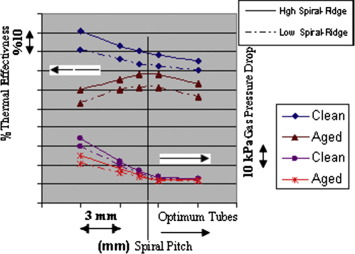Figure 12. Influence of pitch and height of spiral on effectiveness and pressure drop for clean and fouled tubes.

## 4. Effect of proportion of tube flow head diameter to shell diameter

Gas flow equal dispensation into tubes is one of important subjects in EGR cooler entrance head design. Fig. 13 shows circular arrangement for 19 tubes of 3/8 in. in the shell with 63 mm diameter. Tubes are numbered from 1 to 19 to facilitate tubes flow percentage indication in Fig. 14.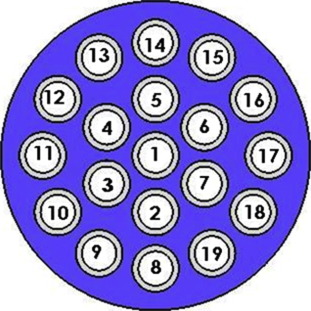Figure 13. Circular arrangement with denoting tubes’ numbers in scope of flow calculation.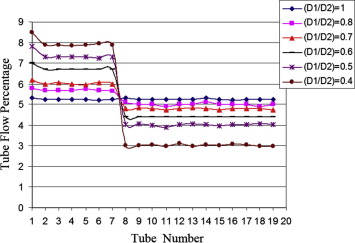Figure 14. Influence of entrance head diameter to shell diameter on the tubes’ flow.

At low proportions of entrance diameter to shell diameter (D1/D2), flow percent decreases at side tubes and as a result, more flow is passed into the centrals. For example, at D1/D2 = 0.4, flow velocity in the central tubes is 35% more than side tubes; this causes pressure reduction at central tubes and cooling water temperature increment near them, which can make local boiling at that section. Local boiling causes cooler vibration and thermal stresses in working time. Also flow rate decrement and subsequently turbulence decrement at side tubes, cause local heat transfer rate reduction. Fluid velocity reduction can prompt sediment increase too. All of these, cause decrement of effectiveness and lifetime of cooler. Fig. 15 shows influence of internal diffuser diameter to shell diameter ratios of 40% and 70%. Temperature increase in the central tubes, because of high flow rate of gas and consequently temperature raise of cooling fluid near them, is apparently visible.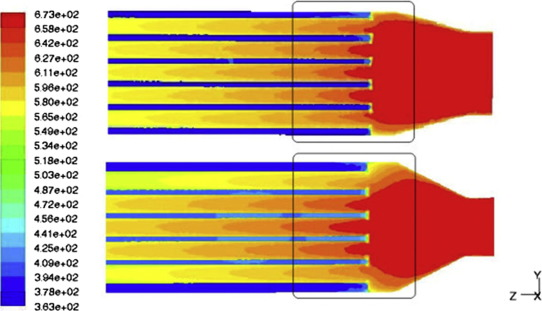Figure 15. Influence of diffuser entrance diameter to shell diameter ratio (40% & 70%).

In other side, if the proportion of D1/D2 be less than before, we will need more length for diffuser to distribute flow appropriately and equally. This will oblige larger cooler length and more pressure-drop in gas side. Based on fittings’ type and EGR tube connectors, with regard to acquired results, suggested ratios of D1/D2 are 60% to 75%. Of course amounts of D1 parameter are restricted regarding tubes’ diameter.

## 5. Cooler design for a sample diesel engine

Cooler design for diesel engine of 4–248 M.F. Tractors, after performance and pollution tests and calculation of maximum quantity of EGR, is done and results are given in Table 1; tests reveal that cold EGR growth, more than 20%, will increase grime amount significantly. With regard to gasoil properties, high percent of brimstone in it, and based on numerous tests, optimum EGR quantity for this engine is 10%; but design is done in a case which works appropriately up to 15%.

Table 1. Design results for normal and optimal cooler.
Optimum cooler Normal cooler
Input gas flow (kg/s) 0.0153 0.015
Input and output gas temperature (K) 473,723 473,723
Tube internal diameter (mm) 7.2 7
Tube external diameter (mm) 9.5 9.5
Number of tubes 19 19
Tube length (mm) 284 410
Pitch proportion 1.25 1.25
Tube pressure drop (Pa) 316 185
Water flow (kg/s) 0.1809 0.1894
Input and output water temperature (°C) 90–85 90–85
Shell internal diameter 63 63

High pressure EGR system, with regard to explained benefits of it, is installed on the engine. Because exhausted EGR gases are extracted for cooling operations before turbocharger, they have a temperature about 500 °C. With respect to tests, temperature of exhausted gases after turbocharger is 70–100 °C less than before. We suppose that thermo-dynamical properties of EGR gases are the same as exhausted engine gases mean specifications, which are combustion productions of sample gasoil with air. The used fuel (gasoil) chemical formulation is C12H26.

Cooling temperature range is very important. In scope of considering brimstone particles effects on the cooling EGR system, some hypothetical and experimental works are done. Researches exhibit that condensation of various brimstone compositions, in every temperature, is dependent on the chemical syntheses of exhausted gases; but dew point of exhausted gases sulfuric acid, by using a fuel with 370 ppm sulfur, is about 130 °C . More temperature decrement causes sulfur and water vapor condensation at backflow gases; consequently sulfuric acid formation causes cooling tubes erosion and deterioration of valves, EGR system connectors, and combustion chamber. Also high temperatures of exhausted gases, impediments appropriate reduction of NOX and grime. With regard to tests, cooler outlet proper temperature is about 170–210 °C.

## 6. Conclusion

Acquired results, for optimum cooler, exhibit weight and dimensions decrement in comparison with typical coolers; it is due to high surface density, effectiveness increment, and equal flow distribution into the tubes. Negative effects of pressure-drop are decreased significantly; moreover, cooler has a high perpetuity against thermal stresses.

One way spiral tube usage, increases cooler thermal effectiveness up to 10% in comparison with typical coolers and because of semi-uniformity of flow in the tubes, using multi way spiral tubes causes pressure-drop reduction; moreover, it does not have high negative influence on the thermal efficiency, and it is the result of heat transfer area increment. Also increment of fluid spinning velocity helps to sediment reduction, self-cleaning, and heat transfer increment.

Pitch size reduction and spiral depth increment, are effective factors at heat transfer and pressure-drop growth.

Simulations, with regard to pitch size, height, number of spirals and sediment effects, are done to ideal and optimal tube design with balance between heat transfer effectiveness and pressure-drop at EGR cooler working range. Entrance diffuser and inlet diameter to shell diameter ratio, in scope of uniform flow distribution, thermal effectiveness increment, and pressure-drop reduction, are investigated. Standard diffuser shape with 60–70% diameter ratios gives best results.

### Document informationPublished on 12/04/17

Licence: Other

### Document Score0

Views 79
Recommendations 0